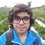# Pre-RMO 2014/16

In a triangle $$ABC$$, let $$I$$ denote the incenter. Let the line $$AI, BI$$ and $$CI$$ intersect the incircle at $$P, Q,$$ and $$R$$, respectively. If $$\angle BAC = 40^\circ$$, what is the value of $$\angle QPR$$ in degrees.

This note is part of the set Pre-RMO 2014Note by Pranshu Gaba
6 years, 8 months ago

This discussion board is a place to discuss our Daily Challenges and the math and science related to those challenges. Explanations are more than just a solution — they should explain the steps and thinking strategies that you used to obtain the solution. Comments should further the discussion of math and science.

When posting on Brilliant:

• Use the emojis to react to an explanation, whether you're congratulating a job well done , or just really confused .
• Ask specific questions about the challenge or the steps in somebody's explanation. Well-posed questions can add a lot to the discussion, but posting "I don't understand!" doesn't help anyone.
• Try to contribute something new to the discussion, whether it is an extension, generalization or other idea related to the challenge.
• Stay on topic — we're all here to learn more about math and science, not to hear about your favorite get-rich-quick scheme or current world events.

MarkdownAppears as
*italics* or _italics_ italics
**bold** or __bold__ bold
- bulleted- list
• bulleted
• list
1. numbered2. list
1. numbered
2. list
Note: you must add a full line of space before and after lists for them to show up correctly
paragraph 1paragraph 2

paragraph 1

paragraph 2

[example link](https://brilliant.org)example link
> This is a quote
This is a quote
    # I indented these lines
# 4 spaces, and now they show
# up as a code block.

print "hello world"
# I indented these lines
# 4 spaces, and now they show
# up as a code block.

print "hello world"
MathAppears as
Remember to wrap math in $$ ... $$ or $ ... $ to ensure proper formatting.
2 \times 3 $2 \times 3$
2^{34} $2^{34}$
a_{i-1} $a_{i-1}$
\frac{2}{3} $\frac{2}{3}$
\sqrt{2} $\sqrt{2}$
\sum_{i=1}^3 $\sum_{i=1}^3$
\sin \theta $\sin \theta$
\boxed{123} $\boxed{123}$

## Comments

Sort by:

Top Newest

Let $\angle IBC= x^\circ$ and $\angle ICB=y^\circ$

$\Rightarrow \angle ABC=2x^\circ \Rightarrow \angle ACB=2y^\circ$ (incenter is the point of concurreny of the angle bisectors)

By apply angle sum, we get

$x^\circ+y^\circ=70^\circ$

$\angle BIC= 180^\circ -(x^\circ +y^\circ)=110^\circ$

$\angle QPR=110^\circ=2\times \angle QPR$ (Angle subtended at the center is double the angle subtended at the circumference)

$\Rightarrow \boxed{\angle QPR=55^\circ}$

- 6 years, 8 months ago

Log in to reply

Thanks :)

- 6 years, 8 months ago

Log in to reply

is it 55 ???

- 6 years, 8 months ago

Log in to reply

Yes it is

- 6 years, 8 months ago

Log in to reply

55

- 6 years, 8 months ago

Log in to reply

How did you solve this problem? I assumed it to be an isosceles triangle. I got the correct answer, but couldn't figure out using a proper method.

- 6 years, 8 months ago

Log in to reply

55

- 6 years, 1 month ago

Log in to reply

40??

- 6 years, 8 months ago

Log in to reply

80??

- 6 years, 8 months ago

Log in to reply

Nooo

- 2 years, 10 months ago

Log in to reply

×

Problem Loading...

Note Loading...

Set Loading...